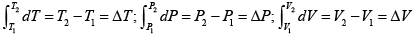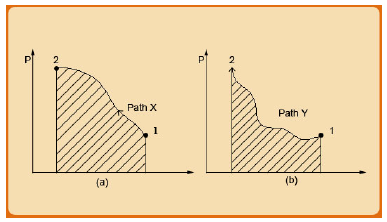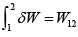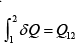Courses

# State and Path Dependent Thermodynamic Variables Civil Engineering (CE) Notes | EduRev

## Civil Engineering (CE) : State and Path Dependent Thermodynamic Variables Civil Engineering (CE) Notes | EduRev

The document State and Path Dependent Thermodynamic Variables Civil Engineering (CE) Notes | EduRev is a part of the Civil Engineering (CE) Course Thermodynamics.
All you need of Civil Engineering (CE) at this link: Civil Engineering (CE)

State and Path Dependent Thermodynamic Variables

Consider a gas at a certain temperature and a pressure within a piston-cylinder assembly (for example, fig. 1.2), which for arguments’ sake we may assume to be isolated. If the piston position is held fixed at this point the gas state is said to be characterized by the temperature and the pressure and its corresponding volume. In its simplest form the relationship between these intensive variables may be described by (say) eqn. 1.12. Consider next that the gas is compressed by application of an extra force on the piston so that it moves inwards into the cylinder. This motion will continue till it reaches a point when the internal gas pressure equals the externally applied pressure on the piston. If there is no further increase in the force applied to the piston, the gas will also attain a new equilibrium state wherein the pressure and temperature would attain a new set of values. If, on the other hand the extra applied pressure is removed and the gas reverts to the earlier state the original temperature and pressure (and, of course volume) is restored. Extending this argument, in general, if the gas is heated or cooled, compressed or expanded, and then returned to its initial temperature and pressure, its intensive properties are restored to their initial values. It is evident, therefore, that such properties do not depend on the past history of the fluid or on the path by which it reaches a given state. They depend only on present state, irrespective of how they are attained. Such quantities are thus defined as state variables. Mathematically, this idea may be expressed as follows:....(1.14)

The changes in the above intensive properties depend only on the initial and final states of the system. They constitute point functions and their differentials are exact.

Let us next consider the case of thermodynamic work as defined by eqn. 1.6. It may be readily evident that if one can depict the exact variation of pressure and volume during a change of state of a system on a two-dimensional P-V graph, the area under the curve between the initial and final volumes equal the work associated with process. This is illustrated in fig. 1.4.Fig. 1.4: Depiction of thermodynamic work on P-V plot

As shown in the above figure the work associated with a thermodynamic process clearly in dependent on the path followed in terms of P and V. It follows that if one were to go from state ‘1’ to ‘2’ by path X and then return to ‘1’ by path Y the work in the two processes would differ and so one would not be giving and taking work out of the system in equal measure. An entity such as P-V work is, therefore, described as a path variable, and therefore is not directly dependent on the state of the system. This is obviously distinctive from the case of state variables such as P and V (and T). Thus, for quantifying work, one cannot write an equation of the same type as (1.12). The more appropriate relation for such variables may be written as:..(1.15)

It may be pointed out that the notation δ is used to depict differential quantum of work in order to distinguish it from the differential quantity of a state variable as in eqn. 1.14. We demonstrate in chapter 3 that, like P-V work, heat transferred between a system and the surrounding is also a path variable and so one may also write:..(1.16)

Heat and work are therefore quantities, and not properties; they account for the energy changes that occur in the system and surroundings and appear only when changes occur in a system. Although time is not a thermodynamic coordinate, the passage of time is inevitable whenever heat is transferred or work is accomplished.

Offer running on EduRev: Apply code STAYHOME200 to get INR 200 off on our premium plan EduRev Infinity!

## Thermodynamics

56 videos|92 docs|33 tests

,

,

,

,

,

,

,

,

,

,

,

,

,

,

,

,

,

,

,

,

,

;